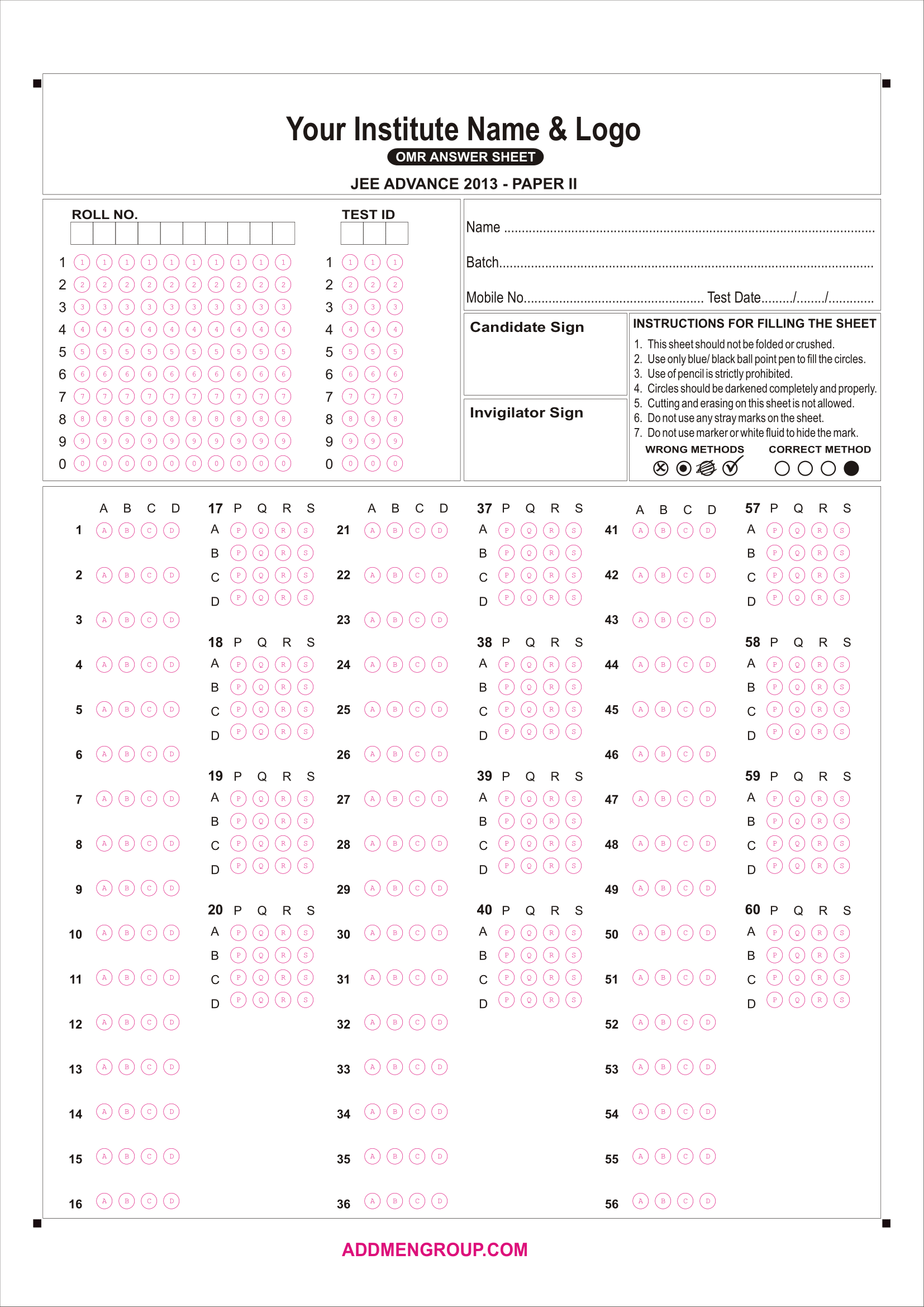# OMR Answer Sheet Design with Matrix Matching Question Blocks

This is an example of IIT JEE Advanced OMR Sheet for Paper 2. In this sheet there are 3 sections for Physics; Chemistry & Math’s each section having 20 questions. Question nos. 1-16 is a single Choice/multiple choice questions having ABCD type of MCQ OMR block.

Question nos. 17-20 are a Matrix matching type of questions with 4 columns (P,Q,R,S) and 4 rows (A,B,C,D).

For Example, Correct Answer to Q 17: A=P; B=R; C=S; D=Q; The answers for this type of Matrix blocks are entered in the following manner: P-R-S-Q